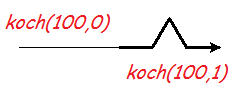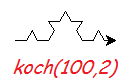# The Koch Snowflake¶

This project draws a fractal curve, with only a few lines of turtle graphics code. It assumes you know about for-loops and functions. And it introduces the computer science idea of recursion.

## The basic unit¶

Start the project by making an empty file `koch.py`. Right-click and open it with IDLE.

We’re going to define a function that either draws a line with a kink in it, or draws a straight line the same length. Which one it does will depend on whether the argument `order` is greater than zero. Type this in the editor, save it (ctrl-S) and run it (F5):

```# Draw a Koch snowflake
from turtle import *

def koch(a, order):
if order > 0:
for t in [60, -120, 60, 0]:
forward(a/3)
left(t)
else:
forward(a)

# Test
koch(100, 0)
pensize(3)
koch(100, 1)
```

The symbol `>` means “greater than”. Be careful about the indenting (the spaces before each line). You should get this:## Recursion¶

This is where your head explodes. You will replace the `forward(a/3)` with another call to `koch`, to draw a kinked line of the same length. That’s a line with 4 parts, …

• and each of those parts has 4 parts,
• and each of those parts has 4 parts,
• and each of those parts has 4 parts, …

Well, not in practice. In practice, the variable `order` goes down by one each time, and when it hits zero, we just draw a line. Change the function `koch`, just a little:

```        for t in [60, -120, 60, 0]:
koch(a/3, order-1)
left(t)
```

When a function calls itself, we say it is recursive. Recursion can solve problems that simple iteration (like a for-loop) cannot.

Save and run. You will get the same two curves as before, because of the test code. But try this in the shell:

```>>> reset()
>>> koch(100, 2)
```

Now each line segment has become a Koch curve. There are 16 little lines.What happens for `koch(100,3)` or `koch(100,4)`? The order 4 curve has 256 tiny lines. One thing that happens is that it starts to take a long time to draw. A call to `speed("fastest")` before you start drawing will help.

## Need for speed¶

The drawing is still too slow once `order` is 6 or more. (The calculation is fast.) The `turtle` module wants to show you every change as it is happening: good when debugging, not so good when you just want the result.

We can delay putting the actions on the screen with the `tracer` function. But then we have to tell it to appear using the `update` function, when we’re ready.

## Looks like snow¶

The snowflake is made from 3 Koch curves. Although there are only 3 “sides”, it has 6-fold symmetry, like a real snowflake.

Delete the test code from your program, and add this program at the end:

```# Choose colours and size
color("sky blue", "white")
bgcolor("black")
size = 400
order = 0

# Ensure snowflake is centred
penup()
backward(size/1.732)
left(30)
pendown()

# Make it fast
tracer(100)
hideturtle()

begin_fill()

# Three Koch curves
for i in range(3):
koch(size, order)
right(120)

end_fill()

# Make the last parts appear
update()
```

Save and run. This should give you a big, white triangle. Adjust `size` so it nicely fills the drawing window when run. Now set `order` to 6 or 7. Run the program and enjoy the result.

How many sides does this have? (Hint: every time the order goes up by one, there are 4 times as many.)